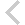FICO Xpress Optimization Examples Repository
 FICO Optimization Community FICO Xpress Optimization HomePortfolio optimization using pandas to calculate covariance

Description
Modeling a small QP problem to perform portfolio optimization using pandas Python library to calculate covariance matrix.

Further explanation of this example: see Chapter 'Python' in the 'Mosel Language Reference Manual'

Source Files
By clicking on a file name, a preview is opened at the bottom of this page.

Data Files

folioqp_pandas.mos

(!*********************************************************
Mosel Example Problems
======================

file folioqp_py.mos

Modeling a small QP problem
to perform portfolio optimization.
-- 1. QP: minimize variance
2. MIQP: limited number of assets --
-- Using Python to calculate covariance matrix --

!!! This example requires an installation of Python 3, see
!!! chapter 'python3' of the 'Mosel Language Reference' for
!!! compatible versions and setup instructions.

(c) 2019 Fair Isaac Corporation
authors: S. Lannez, J. Müller
*********************************************************!)

model "Portfolio optimization with QP/MIQP"
uses "mmxprs", "mmnl"
uses "python3"                      ! Use Python functions

parameters
MAXVAL = 0.3                       ! Max. investment per share
MINAM = 0.5                        ! Min. investment into N.-American values
MAXNUM = 4                         ! Max. number of different assets
TARGET = 9.0                       ! Minimum target yield
end-parameters

declarations
SHARES = 1..10                     ! Set of shares
RISK: set of integer               ! Set of high-risk values among shares
NA: set of integer                 ! Set of shares issued in N.-America
DATES: set of string               ! Historical dates
RET: array(SHARES) of real         ! Estimated return in investment
VAR: array(SHARES,SHARES) of real  ! Variance/covariance matrix of
! estimated returns
OPEN: array(SHARES,DATES) of real  ! Historical share value at market opening
CLOSE: array(SHARES,DATES) of real ! Historical share value at market closing
end-declarations

initializations from "folioqp.dat"
RISK RET NA
end-initializations

! Load historical values to compute the covariance
initializations from "folioqphist.dat"
OPEN CLOSE
end-initializations

! **** Perform some statistics using Python ****

! Import functions from Python script
writeln('Importing pandas and covariance function.')
pyinitpandas
pyrun('folioqp_pandas.py')

! Copy multiple arrays as DataFrame to Python environment.
! It's also possible to use the Python IO driver to set global variables.
pysetdf('share_values', [OPEN, CLOSE], ['open', 'close'])
pyexec('share_values.index.names = ["shares", "dates"]')
pyexec('print(share_values)')

! Print covariance of share value at market openings
writeln("Covariances at market openings:")
pyexec('covariance_of_series(share_values.open)')
! Calculate and retrieve covariance of mean value
writeln("Covariances of mean value of openings and closings:")
pyget('covariance_of_series(share_values.mean(axis=1))', VAR)

declarations
frac: array(SHARES) of mpvar      ! Fraction of capital used per share
end-declarations

! **** First problem: unlimited number of assets ****

! Objective: mean variance
Variance:= sum(s,t in SHARES) VAR(s,t)*frac(s)*frac(t)

! Minimum amount of North-American values
sum(s in NA) frac(s) >= MINAM

! Spend all the capital
sum(s in SHARES) frac(s) = 1

! Target yield
sum(s in SHARES) RET(s)*frac(s) >=  TARGET

! Upper bounds on the investment per share
forall(s in SHARES) frac(s) <= MAXVAL

! Solve the problem
minimize(Variance)

! Solution printing
writeln("With a target of ", TARGET, " minimum variance is ", getobjval)
forall(s in SHARES) writeln(s, ": ", getsol(frac(s))*100, "%")

! **** Second problem: limit total number of assets ****

declarations
buy: array(SHARES) of mpvar       ! 1 if asset is in portfolio, 0 otherwise
end-declarations

! Limit the total number of assets
sum(s in SHARES) buy(s) <= MAXNUM

forall(s in SHARES) do
end-do

! Solve the problem
minimize(Variance)
writeln("With a target of ", TARGET," and at most ", MAXNUM,
" assets,\n minimum variance is ", getobjval)
forall(s in SHARES) writeln(s, ": ", getsol(frac(s))*100, "%")

! Round integer values and resolve
fixglobal(true)
minimize(Variance)
writeln("With all binary variables rounded to the nearest integer:")
forall(s in SHARES) writeln(s, ": ", getsol(frac(s))*100, "%")

end-model

`